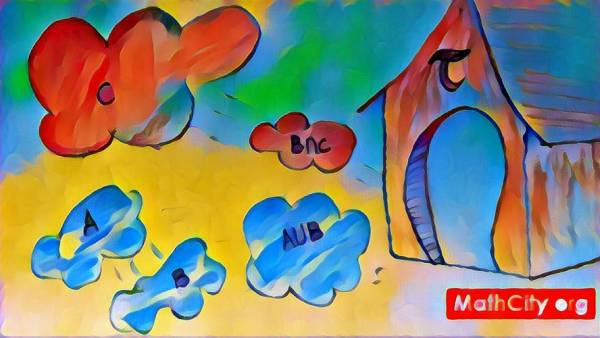# Topology: Handwritten NotesA topological space is a collection of points with a topology-a structure that describes how close two points are to one another. It is a generalisation of Euclidean spaces that makes it possible to investigate boundaries, continuity, and connectivity. A topology is a group of open sets, or subsets, that adhere to certain principles.

A handwritten notes of Topology by Mr. Tahir Mehmood. These notes covers almost every topic which required to learn for MSc mathematics.

 Name Topology: Handwritten Notes Mr. Tahir Mahmood 262 pages Scanned PDF 10.08 mB
• Metric space
• Minkowski's inequality
• Open set
• Closed ball
• Closed set
• Bounded set
• Limit point
• Closure of a set
• Convergence in metric space and complete metric space
• Cauchy sequence
• Bounded sequence
• Nested interval property or Cantor's intersection theorem
• Continuous function
• Topological spaces
• Metric topology, cofinite topology
• Open set
• Closed set
• Closure of a set
• Neighbourhood
• Interior point, exterior point
• Boundary point
• Limit point (with respect to topology)
• Isolated point
• Dense
• Separable set; Countable set
• Base of topology
• Neighbourhood base or local base or base at a point
• Open cover; Lindelof space
• Lindelof theorem
• Relative topology, subspace
• Separation axioms; $T_0$-space
• $T_1$-space
• Subbase; Generation of topologies
• $T_2$-space
• Continuous function (with respect to topologies)
• Product topology
• Convergence of sequence in topological spaces
• Regular space
• Completely regular space
• Compactness in topological spaces
• Homeomorphism
• Countably compact space
• Bolzano Weierstrass property
• Lebesgue number; Big set; Lebesgue covery lemma
• $\varepsilon-$net; Totally bounded
• Connected spaces; Disconnected
• Component
• Totally disconnected
• Separated
• Normed spaced
• Uniformly continuous
• Closed unit ball; Convex set
• Vector space
• Linear combination; Spanning set; Linearly independent
• Linearly dependent
• Linearly independent lemma
• Finite dimensional; Subspace
• Equivalent norms
• Banach space
• Reiz Lemma
• Hilbert spaces; Inner product spaces
• Polarization identity
• Cauchy Schewarz inequality
• Appalonius identity
• Hilbert space; Pythagorian theorem
• Minimizing vector
• Direct sum
• Orthogonal set; Orthonormal set
• Bessel's inequality
• Total orthonormal sets (definition); Parsevel's equality
• Linear Operator; The Kernel or Null space of a linear operator; Continuous linear operator
• Bounded linear operator
• Norm of a bounded lienar operator
• Linear functionals

Please click on View Online to see inside the PDF.

• notes/topology-handwritten-notes